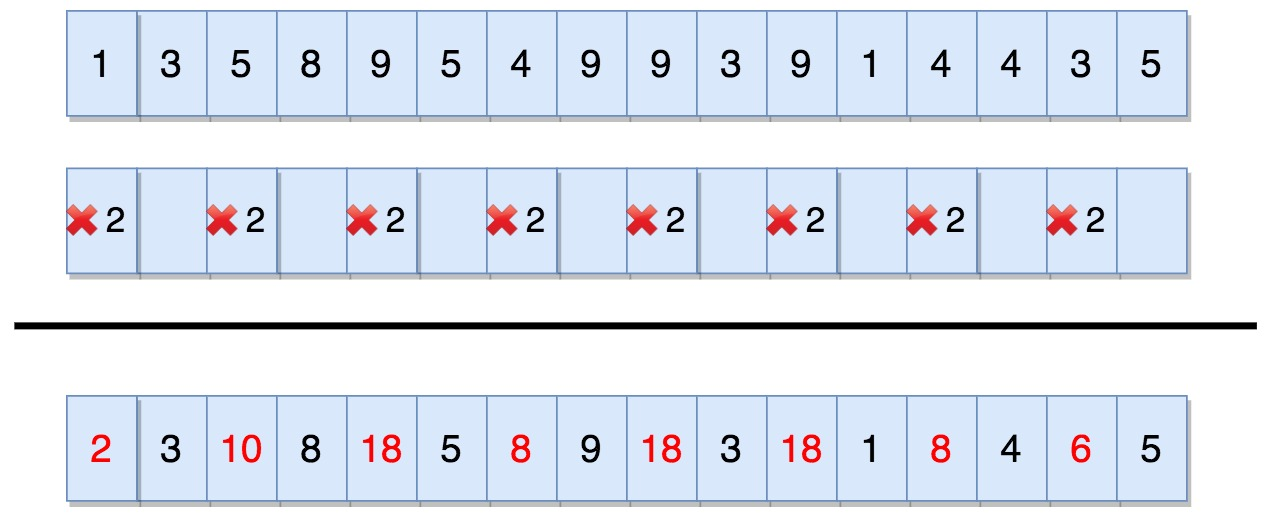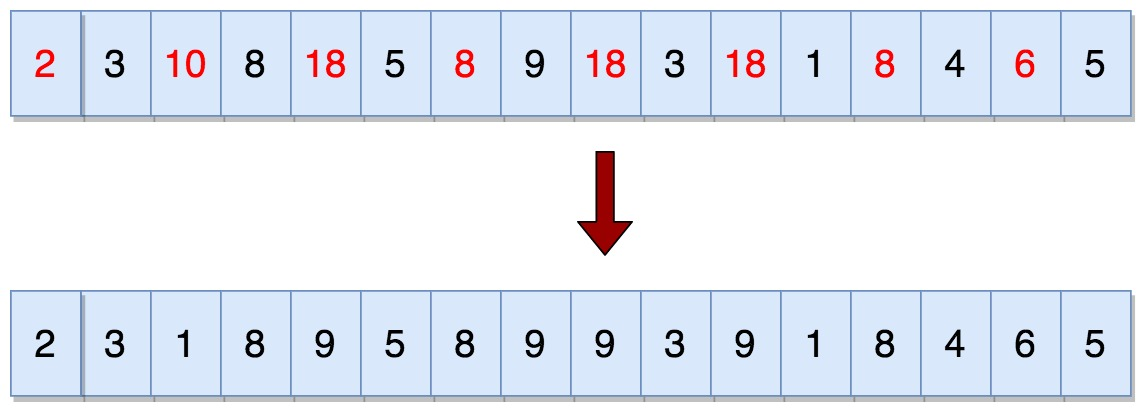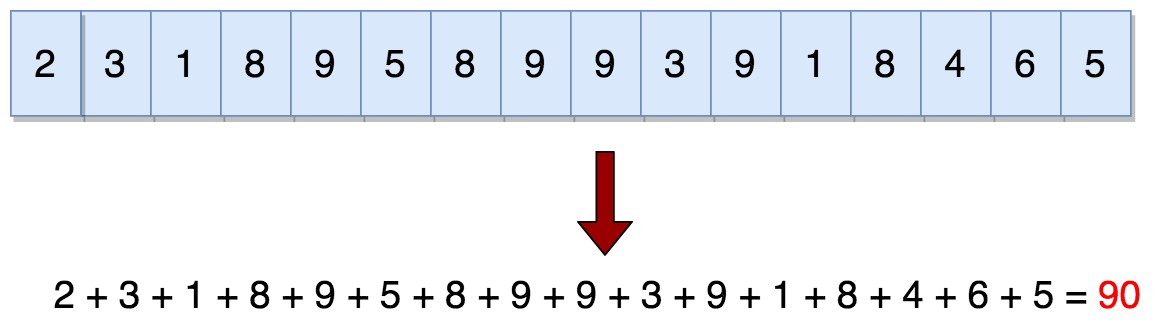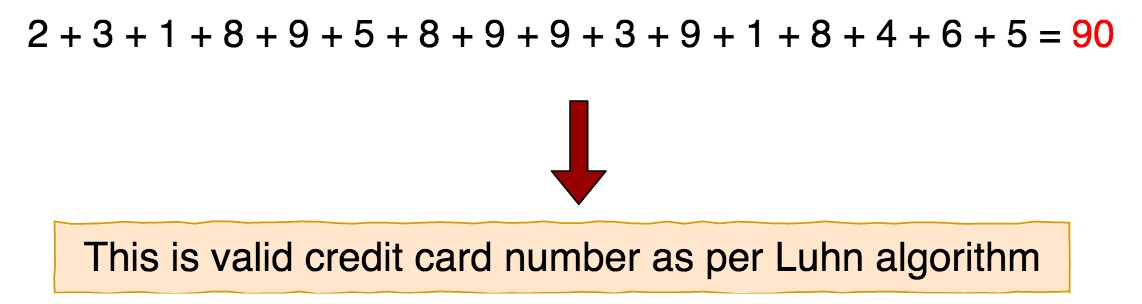# Java credit card validation – Luhn Algorithm in java

In this post, we will see about Luhn Algorithm in java

## Introduction

Luhn algorithm, also known as modulus 10 or mod 10 algorithm, is a simple checksum process for validating various identification numbers such as credit card numbers, Canadian social securities numbers.
This algorithm is designed to protect again mistyped or accidental error rather than malicious attacks. Most credit card companies adopted this algorithm as this was available in the public domain and can be used by anyone.

Here are the steps involved in Luhn Algorithms.

Step 1:
From the rightmost digit, we should double every second digit.Step 2:
When we double the digits and get product in double digits, then we should add digits of the product.Step 3:
Compute the sum of all the digits.Step 4:
If total sum is divisible by 10 i.e. total sum modulo 10 is 0, then number is valid else it is not valid.As 90 mod 10 is 0, hence this is valid credit card number.

## Detailed implementation

Let’s create java program to implement the Luhn algorithm.

Output:

90
1358954993914435 is valid as per luhn algorithm

Here are the steps involved in above java program for luhn algorithm.

1. Convert a String cardNumber to int array cardIntArray for processing
2. Iterate cardIntArray from rightmost side starting from cardIntArray.length-2 with step of i=i-2
3. Mulitply digit by 2
4. If product is greater than 9, sum the product
5. Assign result back to cardIntArray[i]
6. Once the loop is over, compute sum of elements of cardIntArray
7. if sum%10 is 0 then credit card number is valid else not valid as per luhn algorithm

## Using Apache common validation

In case, you don’t want to implement luhn algorithm you can use Apache common validation library and validate credit card number using LuhnCheckDigit.LUHN_CHECK_DIGIT.isValid(creditCardNumber);

You need to add following dependency to pom.xml.

Let’s see with the help of example:

Output:

79927398713 is valid as per luhn algorithm

That’s all about Luhn Algorithm in java